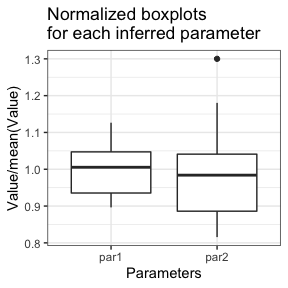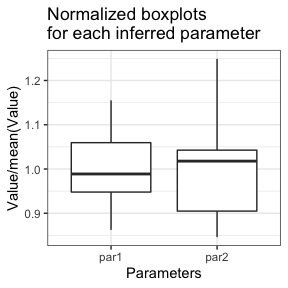# Examples - flimo

## Overview

Flimo (Fixed Landscape Inference MethOd) is a likelihood-free inference method for stochastic models. It is based on simple simulations of the process under study with a specific management of the randomness. This makes it possible to use deterministic optimization algorithms to find the optimal parameters in the sense of summary statistics.

This document presents two small examples to illustrate how the method works.

Inference is possible in R but is more efficient in the Julia language for non-trivial models. This Julia mode uses the Jflimo package available on the git page of the project https://metabarcoding.org/flimo.

## Initial Setup

Only run the first time you load flimo. Installing Julia packages.

julia_setup()
#> Starting Julia ...
#>  TRUE

## Example 1 : Poisson Distribution

Five Poisson variables with parameter = 100 are drawn. We try to find this value by comparing mean of 10 simulated Poisson variables with the observed data. The summary statistic is :

$sumstats(\theta) =\left( \widehat{\mathbb{E}[X|\theta]}-\overline{X^{data}}\right)^2=\left(\frac{1}{n_{sim}}\sum_{i=1}^{n_{sim}}X_i^\theta - \frac{1}{n_{data}}\sum_{i=1}^{n_{data}}X_i^{data}\right)^2$

With the Normal approximation, Poisson distribution is replaced by a Normal distribution with same mean and variance :

$\mathcal{P}(\theta) \leftarrow \mathcal{N}(\mu = \theta, \sigma^2 = \theta)$

#Setup
set.seed(1)

#Create data

Theta_true1 <- 100 #data parameter
n1 <- 5 #data size

simulator1 <- function(Theta, n){
#classical random simulator
rpois(n, lambda = Theta)
}

data1 <- simulator1(Theta_true1, n1)

#Simulations with quantiles
#See README to know how to build this simulator

ndraw1 <- n1 #number of random draws for one simulation

check_simulator(simulator1, ndraw1, 0, 200)
#>  FALSE

simulatorQ1 <- function(Theta, quantiles){
qpois(quantiles, lambda = Theta)
}
check_simulator(simulatorQ1, ndraw1, 0, 200)
#>  TRUE

#With Normal approximation
simulatorQ1N <- function(Theta, quantiles){
qnorm(quantiles, mean = Theta, sd = sqrt(Theta))
}

check_simulator(simulatorQ1N, ndraw1, 0, 200)
#>  TRUE

#Quantile-simulator with Normal approximation

#First simulations

Theta11 <- 50
Theta21 <- 200

nsim1 <- 10

quantiles1 <- matrix(runif(ndraw1*nsim1), nrow = nsim1)

#just one simulation
simulatorQ1(Theta11, quantiles1[1,])
#>  49 43 52 45 49
#Same value :
simulatorQ1(Theta11, quantiles1[1,])
#>  49 43 52 45 49

#independent values :
simulatorQ1(Theta11, quantiles1[2,])
#>  43 55 61 54 50

#Matrix of nsim simulations

simu11 <- simulatorQ1(Theta11, quantiles1)
simu21 <- simulatorQ1(Theta21, quantiles1)

#Sample Comparison : summary statistics

sumstats1 <- function(simulations, data){
#simulations : 2D array
#data : 1D array
mean_simu <- mean(rowMeans(simulations))
mean_data <- mean(data)
(mean_simu-mean_data)^2
}
#Plot objective function

#Objective by parameter :
plot_objective(ndraw1, nsim1, data1, sumstats1, simulatorQ1, lower = 0, upper = 200)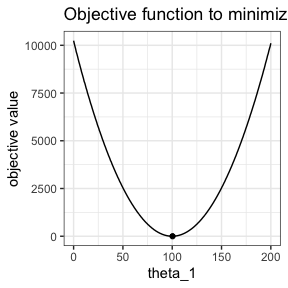#Objective with Normal approximation :

plot_objective(ndraw1, nsim1, data1, sumstats1, simulatorQ1N, lower = 0, upper = 200)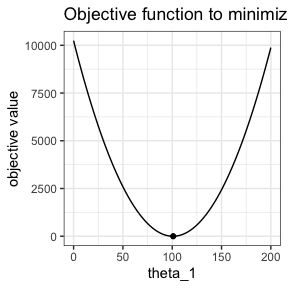#both plots
#We use same quantiles for both

quantiles1 <- matrix(runif(ndraw1*nsim1), nrow = nsim1)

p <- plot_objective(NULL, NULL, data1, sumstats1, simulatorQ1,
lower = 0, upper = 200, quantiles = quantiles1)
plot_objective(NULL, NULL,
data1, sumstats1, simulatorQ1N,
lower = 0, upper = 200,
visualize_min = FALSE,
add_to_plot = p, quantiles = quantiles1)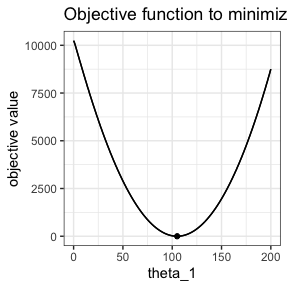#Locally, Poisson quantiles and then objective function are constant by pieces
#To compare with normal approximation

p <- plot_objective(NULL, NULL, data1, sumstats1,
simulatorQ1, lower = 71, upper = 72,
visualize_min = FALSE, quantiles = quantiles1)

plot_objective(ndraw1, nsim1, data1, sumstats1, simulatorQ1N,
lower = 71, upper = 72, visualize_min = FALSE, add_to_plot = p,
quantiles = quantiles1)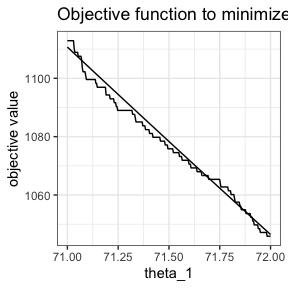Flimo method is illustrated below:

nsimplot <- 5
quantilesplot <- matrix(runif(ndraw1*nsimplot), nrow = nsimplot)

intern_obj <- function(Theta) flimobjective(Theta, quantilesplot, data1, sumstats1, simulatorQ1)

intern_objN <- function(Theta) flimobjective(Theta, quantilesplot, data1, sumstats1, simulatorQ1N)

intern_objRand <- function(Theta){
sim <- rpois(nsim1, Theta)
(mean(sim)-mean(data1))^2
}

x <- c(seq(0, 200, length.out = 1e4), seq(70.5, 72.5, length.out = 1e4))
y <- sapply(x, intern_obj)
yN <- sapply(x, intern_objN)

xR <- seq(0, 200, length.out = 2e3)
yR <- sapply(xR, intern_objRand)

df <- data.frame(x = x, y = y, Method = "Fixed Landscape")
df <- rbind(df, data.frame(x = x, y = yR, Method = "Naive computation"))
df <- rbind(df, data.frame(x = x, y = yN, Method = "Fixed Landscape\nwith Normal Approximation"))

ggplot()+
geom_line(aes(xR,yR), color = "grey")+
geom_line(aes(x,y), color = "blue")+
ggtitle("Inference for a Poisson distribution : value of objective")+
labs(x = "Theta", y = "J(Theta)")+
theme(legend.position='none',
plot.title = element_text(hjust = 0.5))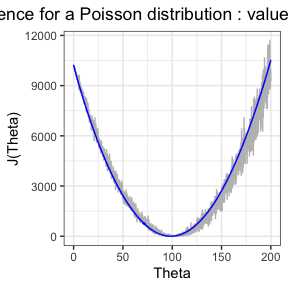# ggsave("../../manuscript/Figures/poisson.png", dpi = 350, units = "mm", width = 180, height = 150)

x1 <- 71
x2 <- x1+1
xin <- x[which(x<=x1)[length(which(x<=x1))]]
yax <- max(y[which(x<=x1)[length(which(x<=x1))]], yN[which(x<=x1)[length(which(x<=x1))]])
xax <- x[which(x>=x2)]
yin <- min(y[which(x>=x2)], yN[which(x>=x2)])

ggplot()+
geom_line(aes(x,y), color = "blue")+
geom_line(aes(x,yN), color = "red")+
ggtitle("Inference for a Poisson distribution : value of objective")+
labs(x = "Theta", y = "J(Theta)")+
theme(legend.position='none',
plot.title = element_text(hjust = 0.5))+
coord_cartesian(xlim = c(xin, xax), ylim = c(yin, yax))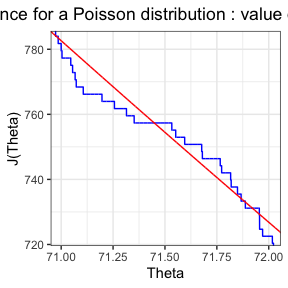# ggsave("../../manuscript/Figures/poisson_zoom.png", dpi = 350, units = "mm", width = 180, height = 150)

There are optimization issues in R for normalized process and Theta close to 0. Lower bound set to 1.

#Optimization with normal approximation of Poisson distribution

#First mode : full R
#default mode

system.time(optim1R <- flimoptim(data1, ndraw1, sumstats1, simulatorQ1N,
ninfer = 10,
nsim = nsim1,
lower = 1, upper = 1000,
randomTheta0 = TRUE))
#> utilisateur     système      écoulé
#>       0.022       0.001       0.022
optim1R
#> optimization Result
#> Mode : R
#> method : L-BFGS-B
#> Number of inferences : 10
#> Convergence : 10/10
#> Mean of minimizer :
#> 101.105782785824
#> Best minimizer :
#> 101.358828149557
#> Reached minima : from 3.79036936450385e-24 to 1.49222794632798e-19
#> Median time by inference 0.00188493728637695
#> optimization Result
#> Mode : R
#> method : L-BFGS-B
#> Number of inferences : 10
#> Convergence : 10/10
#> Mean of minimizer :
#> 101.105782785824
#> Best minimizer :
#> 101.358828149557
#> Reached minima : from 3.79036936450385e-24 to 1.49222794632798e-19
#> Median time by inference 0.00188493728637695
summary(optim1R)
#> $Mode #>  "R" #> #>$method
#>  "L-BFGS-B"
#>
#> $number_inferences #>  10 #> #>$number_converged
#>  10
#>
#> $minimizer #> par1 #> Min. : 99.11 #> 1st Qu.: 99.95 #> Median :101.52 #> Mean :101.11 #> 3rd Qu.:102.01 #> Max. :102.62 #> #>$minimum
#>      Min.   1st Qu.    Median      Mean   3rd Qu.      Max.
#> 3.790e-24 2.638e-22 2.882e-22 1.559e-20 6.127e-22 1.492e-19
#>
#> $median_time_inference #>  0.001884937 attributes(optim1R) #>$names
#>   "mode"      "method"    "AD"        "minimizer" "minimum"   "converged"
#>   "initial_x" "f_calls"   "g_calls"   "message"   "time_run"
#>
#> $class #>  "flimo_result" plot(optim1R)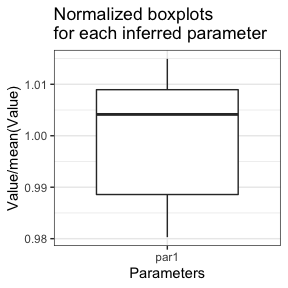#Second mode : full J #Optimization with Automatic Differentiation #Warning : you need to translate sumstats and simulatorQ to Julia #Both of these names for the Julia functions are mandatory ! julia_simulatorQ1N <-" function simulatorQ(Theta, quantiles) quantile.(Normal.(Theta, sqrt.(Theta)), quantiles) end " julia_sumstats1 <-" function sumstats(simulations, data) (mean(mean(simulations, dims = 2))-mean(data))^2 end " #Most accurate config for complex problems : IPNewton with AD if (juliaSetupOk()){ system.time(optim1JAD <- flimoptim(data1, ndraw1, julia_sumstats1, julia_simulatorQ1N, ninfer = 10, nsim = nsim1, lower = 0, upper = 1000, randomTheta0 = TRUE, mode = "Julia", load_julia = TRUE)) optim1JAD summary(optim1JAD) attributes(optim1JAD) plot(optim1JAD) #IPNewton without AD system.time(optim1J <- flimoptim(data1, ndraw1, julia_sumstats1, julia_simulatorQ1N, ninfer = 10, nsim = nsim1, lower = 0, upper = 1000, randomTheta0 = TRUE, mode = "Julia", AD = FALSE)) optim1J summary(optim1J) attributes(optim1J) plot(optim1J) #Brent system.time( optim1JBrent <- flimoptim(data1, ndraw1, julia_sumstats1, julia_simulatorQ1N, ninfer = 10, nsim = nsim1, lower = 0, upper = 1000, randomTheta0 = TRUE, mode = "Julia", method = "Brent") ) optim1JBrent summary(optim1JBrent) attributes(optim1JBrent) plot(optim1JBrent) }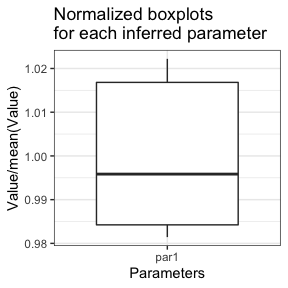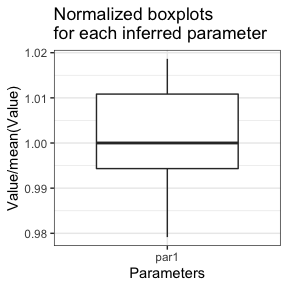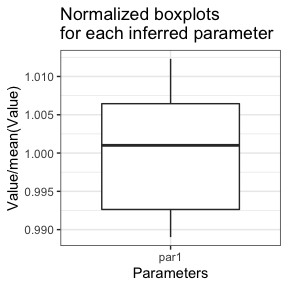## Example 2 : Normal Distribution Five normal variables with mean = 0 and sd = 1 are drawn. We try to find these mean/sd values by comparing mean and sd of 10 simulated normal variables with the observed data. The summary statistic is : $sumstats(\theta) =\left( \widehat{\mathbb{E}[X|\theta]}-\overline{X^{data}}\right)^2 + \left( \widehat{\sigma(X|\theta)}-\sigma(X^{data})\right)^2$ #Setup set.seed(1) #Create data Theta_true2 <- c(3, 2) #data parameter n2 <- 5 #data size simulator2 <- function(Theta, n){ #classical random simulator rnorm(n, mean = Theta, sd = Theta) } data2 <- simulator2(Theta_true2, n2) #Simulations with quantiles #See README to know how to build this simulator simulatorQ2 <- function(Theta, quantiles){ qnorm(quantiles, mean = Theta, sd = Theta) } ndraw2 <- 5 check_simulator(simulatorQ2, ndraw2, c(0, 0), c(10, 10)) #>  TRUE check_simulator(simulator2, ndraw2, c(0, 0), c(10, 10)) #>  FALSE sumstats2 <-function(simulations, data){ mean_simu <- mean(rowMeans(simulations)) mean_data <- mean(data) sd_simu <-mean(apply(simulations, 1, sd)) sd_data <- sd(data) (mean_simu-mean_data)^2+(sd_simu-sd_data)^2 } nsim2 <- 10  plot_objective(ndraw2, nsim2, data2, sumstats2, simulatorQ2, index = 1, other_param = c(1, 2, 10), lower = -5, upper = 10)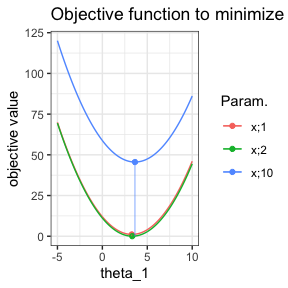#Optimization #First mode : full R #default mode optim2R <- flimoptim(data2, ndraw2, sumstats2, simulatorQ2, ninfer = 10, nsim = nsim2, lower = c(-5, 0), upper = c(10, 10), randomTheta0 = TRUE) optim2R #> optimization Result #> Mode : R #> method : L-BFGS-B #> Number of inferences : 10 #> Convergence : 10/10 #> Mean of minimizer : #> 3.20567648680977 2.11520187608635 #> Best minimizer : #> 3.14574674163785 1.97643436033205 #> Reached minima : from 0 to 3.8290401805314e-14 #> Median time by inference 0.00757956504821777 #> optimization Result #> Mode : R #> method : L-BFGS-B #> Number of inferences : 10 #> Convergence : 10/10 #> Mean of minimizer : #> 3.20567648680977 2.11520187608635 #> Best minimizer : #> 3.14574674163785 1.97643436033205 #> Reached minima : from 0 to 3.8290401805314e-14 #> Median time by inference 0.00757956504821777 summary(optim2R) #>$Mode
#>  "R"
#>
#> $method #>  "L-BFGS-B" #> #>$number_inferences
#>  10
#>
#> $number_converged #>  10 #> #>$minimizer
#>       par1            par2
#>  Min.   :2.975   Min.   :1.681
#>  1st Qu.:3.044   1st Qu.:1.948
#>  Median :3.130   Median :2.116
#>  Mean   :3.206   Mean   :2.115
#>  3rd Qu.:3.211   3rd Qu.:2.276
#>  Max.   :3.820   Max.   :2.536
#>
#> $minimum #> Min. 1st Qu. Median Mean 3rd Qu. Max. #> 0.000e+00 0.000e+00 0.000e+00 3.830e-15 1.000e-18 3.829e-14 #> #>$median_time_inference
#>  0.007579565
plot(optim2R, pairwise_par = TRUE, hist = TRUE, par_minimum = TRUE)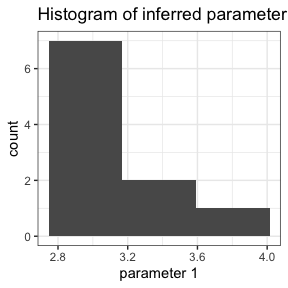#> Warning: Transformation introduced infinite values in continuous y-axis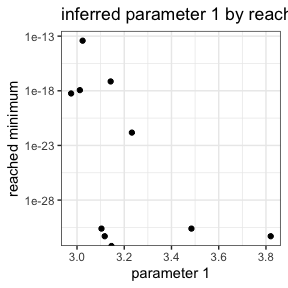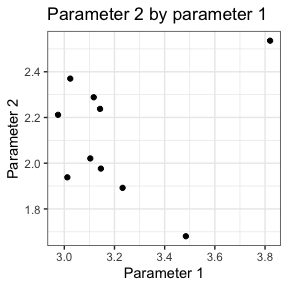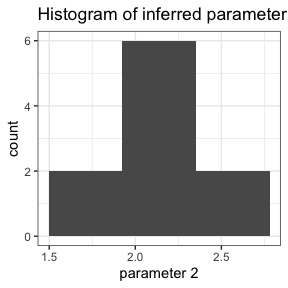#> Warning: Transformation introduced infinite values in continuous y-axis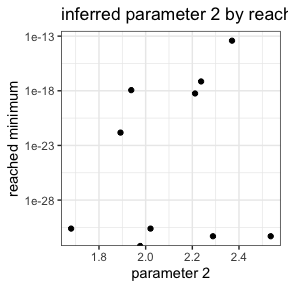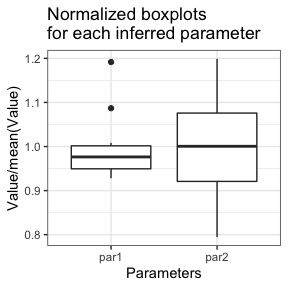#Second mode : full Julia
#Optimization with Automatic Differentiation
#Warning : you need to translate sumstats and simulatorQ to Julia
#Both of these names for the Julia functions are mandatory !

if (juliaSetupOk()){
julia_simulatorQ2 <-"
function simulatorQ(Theta, quantiles)
quantile.(Normal.(Theta, Theta), quantiles)
end
"

julia_sumstats2 <-"
function sumstats(simulations, data)
(mean(mean(simulations, dims = 2))-mean(data))^2+
(mean(std(simulations, dims = 2))-std(data))^2
end
"

#Most accurate config for complex problems : IPNewton with AD
optim2JAD <- flimoptim(data2, ndraw2, julia_sumstats2, julia_simulatorQ2,
ninfer = 10, nsim = nsim2,
lower = c(-5, 0), upper = c(10, 10),
randomTheta0 = TRUE,
mode = "Julia")

}